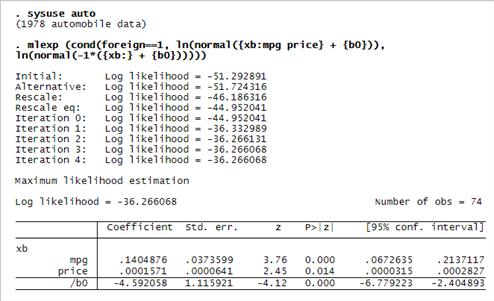HIGHLIGHTS

• Specify log-likelihood function interactively
• Optionally specify first derivatives
• Robust SEs to relax distributional assumptions
• Cluster–robust SEs for correlated data
• Linear and nonlinear postestimation hypotheses tests

SHOW ME

Maximization of user-specified likelihood functions has long been a hallmark of Stata, but you have had to write a program to calculate the log-likelihood function. Now it is even easier. The only requirements are that you be able to write the log likelihood for individual observations and that the log likelihood for the entire sample be the sum of the individual values.

Stata can fit probit models, but let’s write our own.

The log-likelihood function for probit is

```LL(y) = ln(normal(x'b))   if  y==1
= ln(normal(-xb))       y==0```

To fit a model of outcome foreign on mpg and price, we type